﻿ 劳动力回流的要素配置与产业结构优化——兼论“东北困境”与“东北药方”
 上海财经大学学报2018, Vol. 20Issue (3): 29-430

文章信息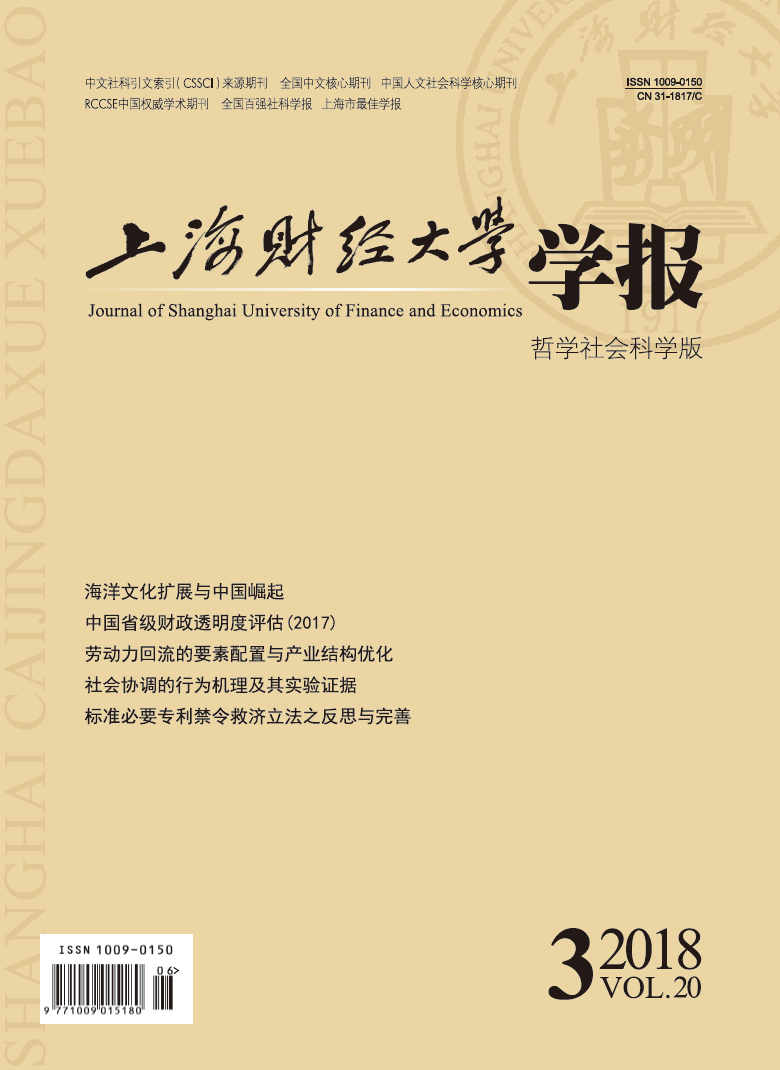上海财经大学2018年20卷第3期

Sun Wei, Liu Zhichao

Factor Allocation and Industrial Structure Optimization in Labor Return Migration: Also Focus on “Northeast Dilemma” and “Northeast Prescription”

Journal of Shanghai University of Finance and Economics, 2018, 20(3): 29-43.

文章历史《上海财经大学学报》
2018第20卷第3期

1.吉林大学　数量经济研究中心，吉林　长春　130012;
2.吉林大学　商学院，吉林　长春　130012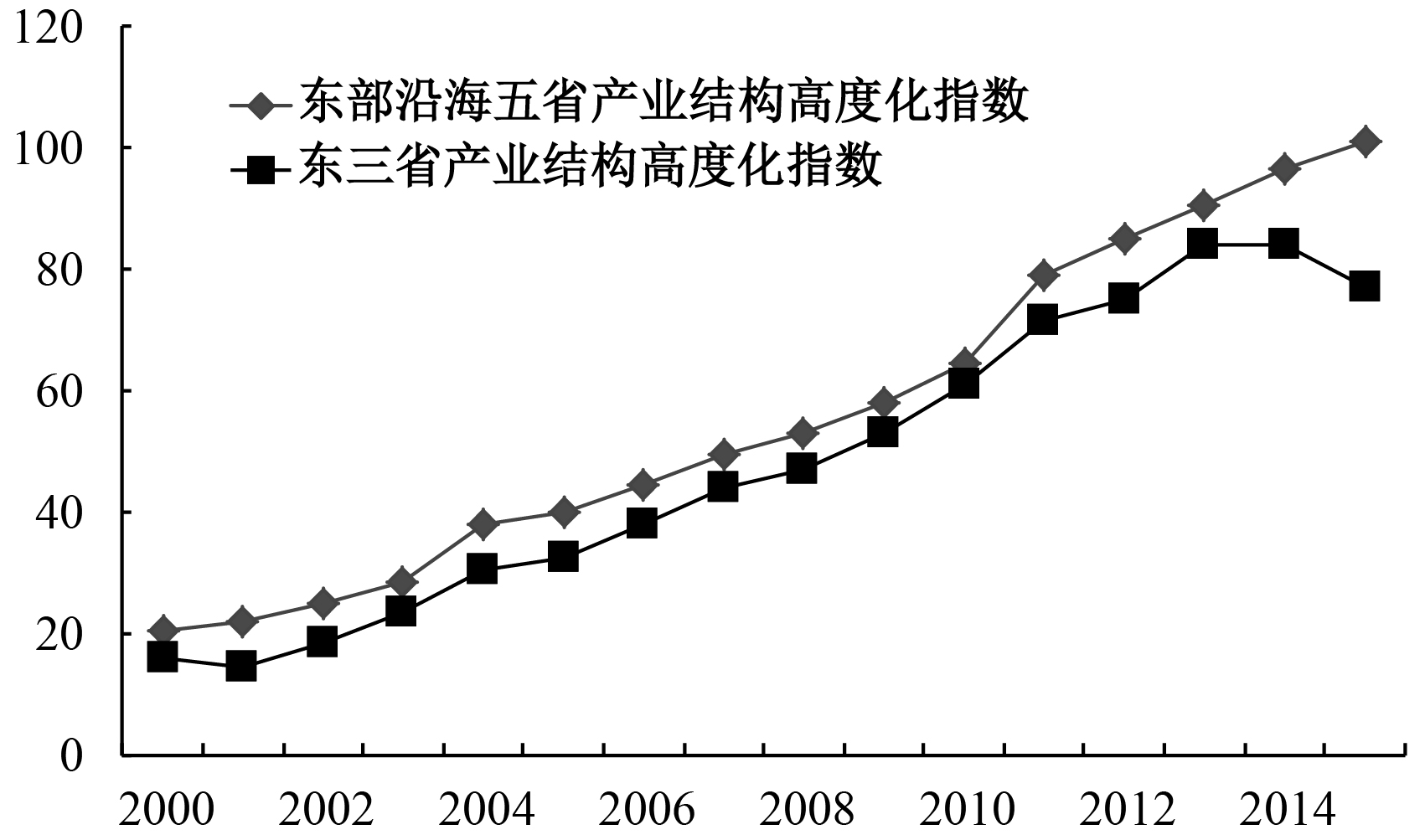图 1 省际产业结构高度化指数演化趋势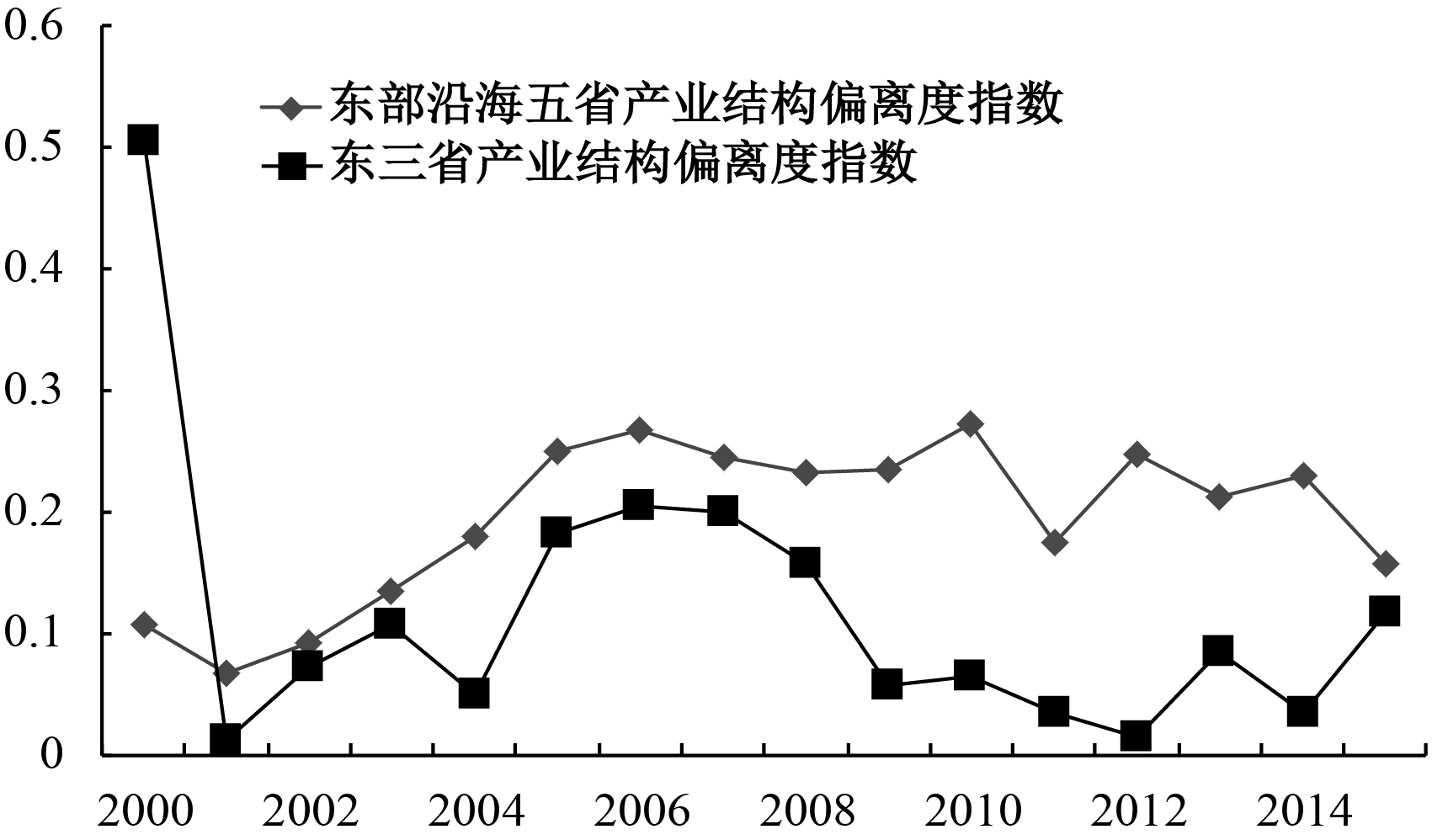图 2 省际产业结构偏离度演化趋势

1.劳动力回流选择与劳动要素供给

 $M = f\left[ {\int_0^n {\left[ {\rho \left( t \right){W_\mu }\left( t \right) - {W_\gamma }\left( t \right)} \right]} {e^{ - rt}}dt - C\left( 0 \right)} \right]$ (1)

2.厂商的资本要素投入选择

 $ro{c_o} = \frac{{{R_o}\left( {{L_o} - \Delta {L_o}} \right)}}{{{K_o}}}$ (2)
 $ro{c_{rm}} = \frac{{{R_{rm}}\left( {{L_{rm}} + \Delta {L_{rm}}} \right)}}{{{K_{rm}}}}$ (3)

(二) 基于国有企业和非国有企业划分的产业结构优化机理分析

 $SH = \sum\limits_{i = 1}^n {\left( {{{{Y_i}} /Y}} \right)\left( {{{L{P_i}} / {LP}}} \right)}$ (4)
 $SD = \sum\limits_{i = 1}^n {\left( {{{{Y_i}} / Y}} \right)\left| {\frac{{\left( {{{{Y_i}} /{{L_i}}}} \right)}}{{{Y / L}}} - 1} \right|}$ (5)

 $SH = \frac{{{{Y_1^2} / {{L_1}}}}}{{{{{Y^2}} / L}}} + \frac{{{{Y_2^2}/ {{L_2}}}}}{{{{{Y^2}} / L}}}$ (6)
 $SD = \left( {{{{Y_1}} / Y}} \right)\left| {\frac{{\left( {{{{Y_1}} / {{L_1}}}} \right)}}{{{Y / L}}} - 1} \right| + \left( {{{{Y_2}} / Y}} \right)\left| {\frac{{\left( {{{{Y_2}} / {{L_2}}}} \right)}}{{{Y / L}}} - 1} \right|$ (7)

 $\ln {Y / L} = \ln A + \alpha \ln {K / L} + \left( {\alpha + \beta - 1} \right)\ln L$ (8)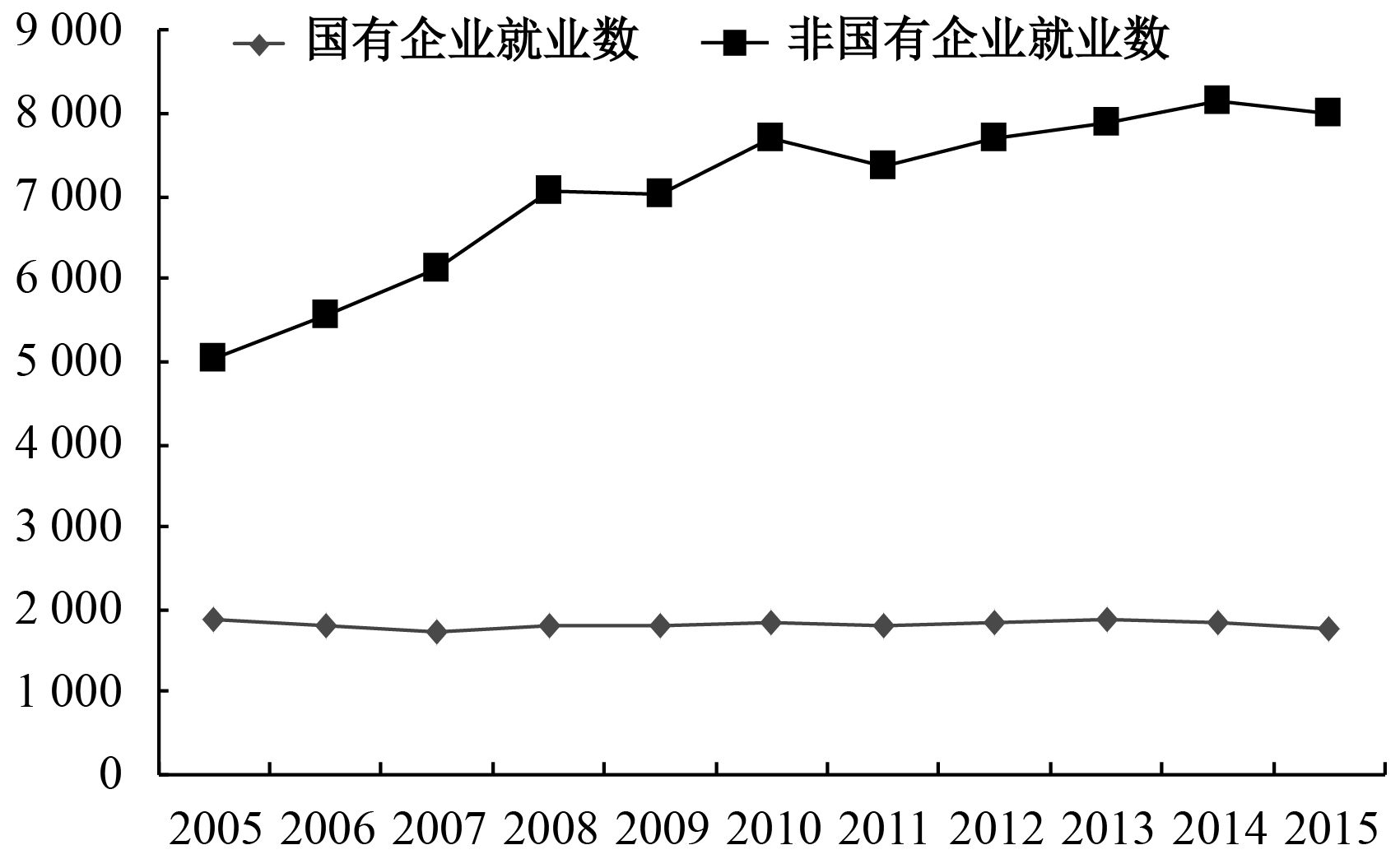图 3 就业人数演化趋势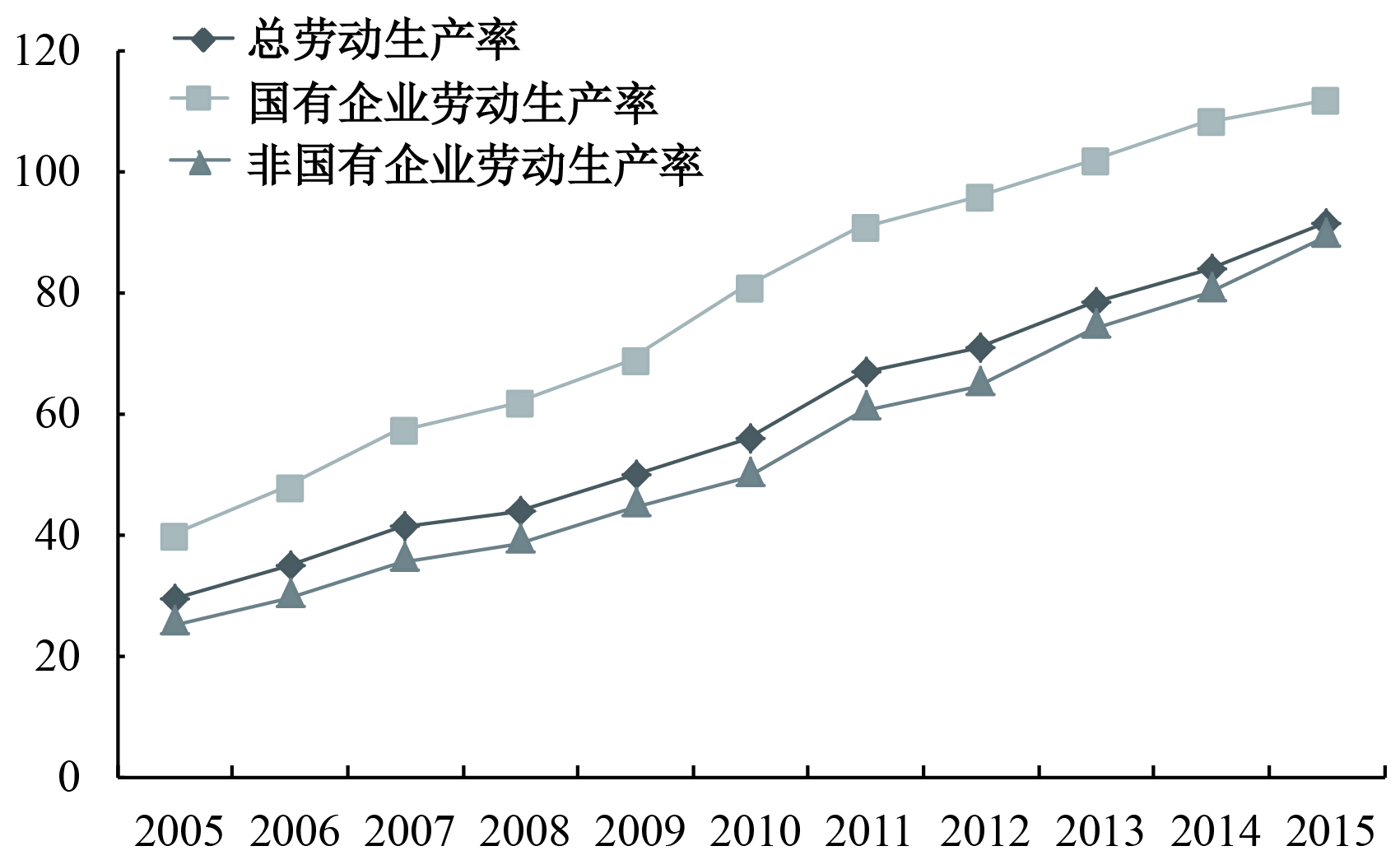图 4 劳动生产率演化趋势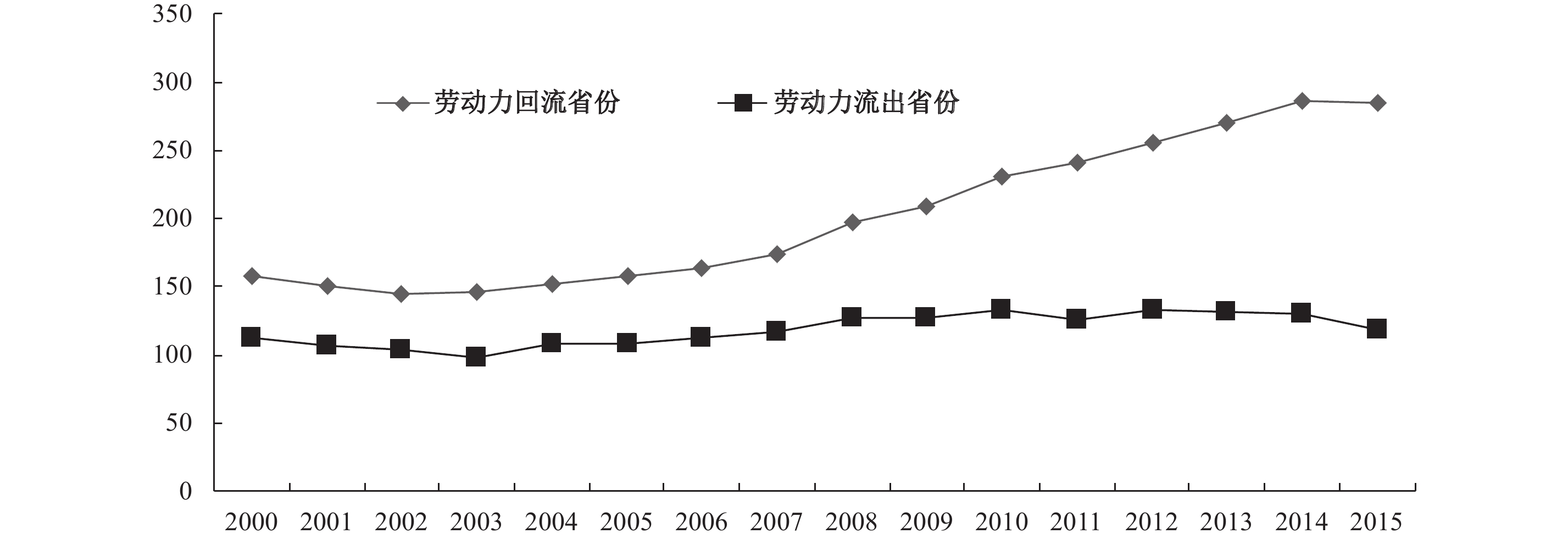图 5 劳动力迁徙省份劳动要素存量均值

(二) 工业劳动力回流机制研究

 $M = f\left[ {\Delta W < q,\Delta W {\text{≥}} q,\Delta R,CL,IS} \right]$ (9)

 模型 F-统计值 Prob BS次数 临界值 1% 5% 10% 单一门限模型 13.866 0.110 1 000 25.098 17.686 14.201 双重门限模型 23.578** 0.025 1 000 27.030 20.646 16.658 注：***、**和*分别代表在 1%、5%和 10%水平上显著。下同。

 门限估计值 95%置信区间 –0.312 [–0.369，–0.187] –1.077 [–1.123，–0.942]

 $M = \delta + {\beta _1}D{V_1}\Delta W + {\beta _2}\Delta W + {\beta _3}D{V_2}\Delta W + {\beta _4}\Delta R + {\beta _5}CL + {\beta _6}IS$ (10)
 $D{V_1} = \left\{ {\begin{array}{*{20}{c}}1,& {\Delta W {\text{≥}} - 0.312}\\0,& {\quad \quad \;\quad \;\;{\text{其他}}}\end{array}} \right. ，\quad\;D{V_2} = \left\{ {\begin{array}{*{20}{c}}1,& {\Delta W {\text{≤}} - 1.077}\\0,& {\quad \quad \;\;\;\quad {\text{其他}}}\end{array}} \right.$

 $M$ 系数 T值 常数项 –0.004 88*** –3.98 $\Delta W$ （ $\Delta W {\text{≥}} - 0.312$ ） 0.003 73*** 4.04 $\Delta W$ –0.003 69*** –5.11 $\Delta W$ （ $\Delta W < - 1.077$ ） 0.002 17*** 4.54 $\Delta R$ 0.000 35*** 4.67 $C$ –0.000 95** –2.05 $IS$ 0.012 44*** 3.71 样本数 368 F值 19.34*** R2 0.255 0

(三) 劳动力回流框架下的资本回报率演化机制研究

 $\ln roc$ 流出省份 $\ln roc$ 回流省份 系数 T值 系数 T值 常数项 –2.21* –1.76 常数项 –3.78*** –8.43 $\ln L$ （ $year < 2013$ ） 1.07*** 3.41 $\ln L$ （ $year < 2003$ ） 1.07*** 11.46 $\ln L$ （ $year {\text{≥}} 2013$ ） 1.00*** 3.26 $\ln L$ （ $year {\text{≥}} 2003$ ） 1.23*** 14.07 样本数 174 样本数 192 F值 6.84*** F值 363.87*** R2 0.78 R2 0.80

 原假设 F值 Prob F H0： $\ln L$ （ $year < 2003$ ）= $\ln L$ （ $year {\text{≥}} 2003$ ） 185.36 0.00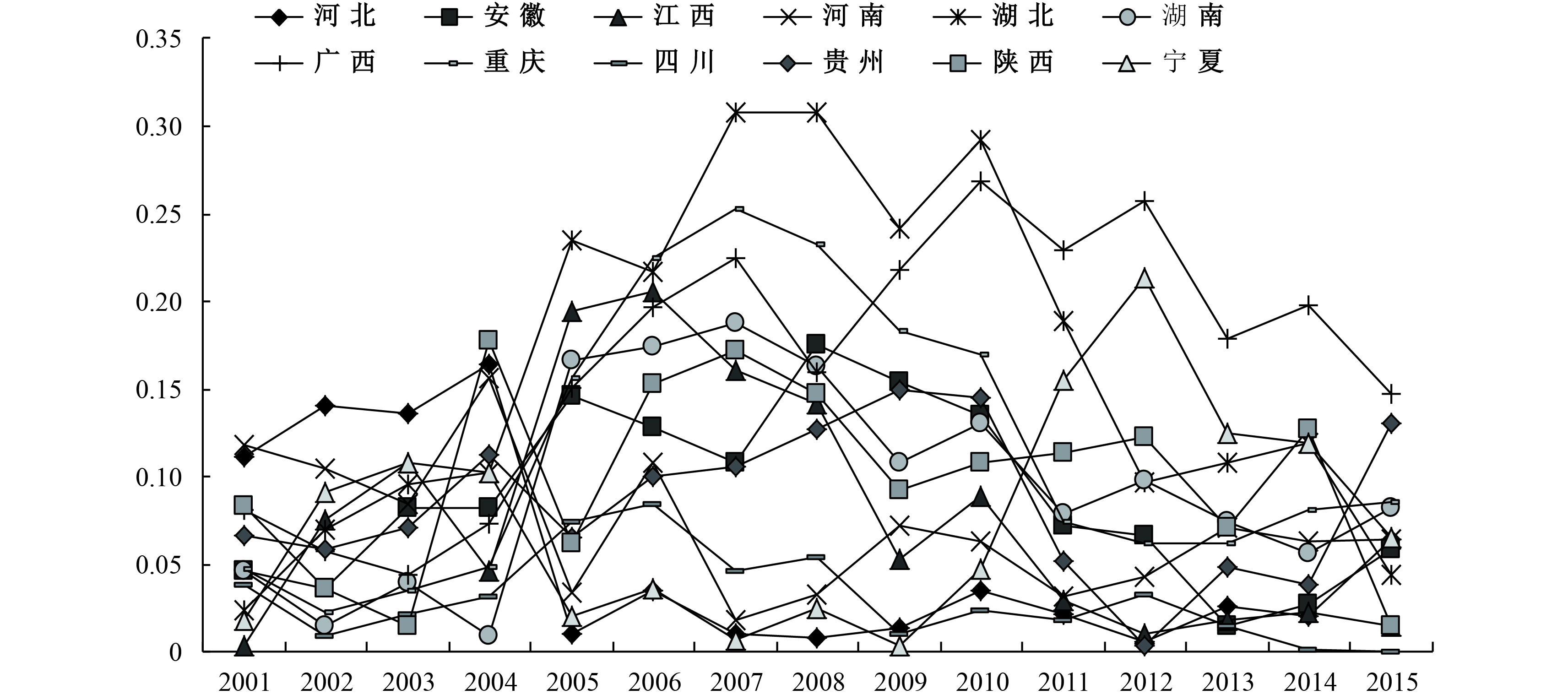图 6 回流区域产业结构偏离度趋势图 7 流出区域产业结构偏离度趋势图 8 回流区域产业结构高度化趋势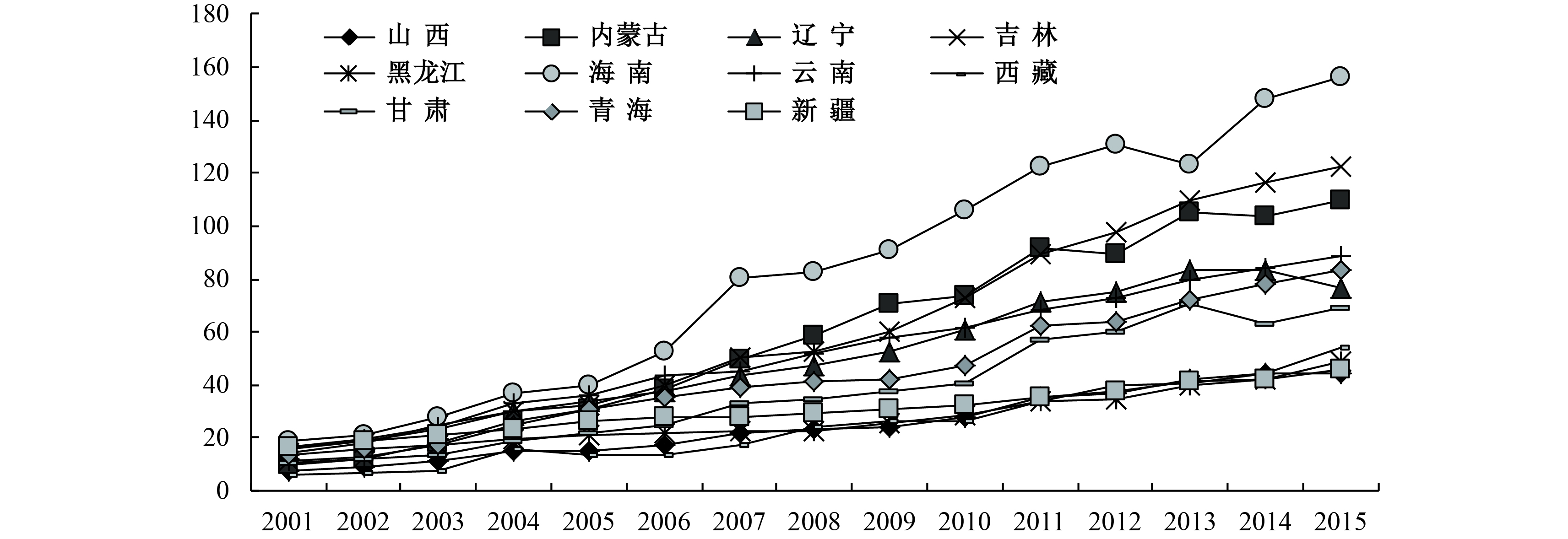图 9 流出区域产业结构高度化趋势
(二) 劳动力回流框架下的产业结构演化趋势研究

 $SH$ 流出省份 SH 回流省份 系数 T值 系数 T值 常数项 –88.81*** –2.82 常数项 –205.48*** –7.82 $\ln L$ （ $year < 2013$ ） 18.50*** 2.81 $\ln L$ （ $year < 2003$ ） 34.53*** 7.41 $\ln L$ （ $year {\text{≥}} 2013$ ） 19.88*** 3.05 $\ln L$ （ $year {\text{≥}} 2003$ ） 33.86*** 7.02 $State - owned\;Y\;ratio$ –67.44*** –7.32 $State - owned\;Y\;ratio$ –27.74*** –2.68 $\ln k$ 28.20*** 12.06 $\ln k$ 30.18*** 14.32 样本数 176 样本数 192 F值 25.61 F值 11.64 R2 0.81 R2 0.94

 $SD$ 流出省份 SD 回流省份 系数 T值 系数 T值 常数项 4.07*** 6.14 常数项 1.79*** 3.87 $\ln L$ （ $year < 2013$ ） –0.39*** –2.78 $\ln L$ （ $year < 2003$ ） –0.19** –2.33 $\ln L$ （ $year {\text{≥}} 2013$ ） –0.38*** –2.74 $\ln L$ （ $year {\text{≥}} 2003$ ） –0.21** –2.49 $State - owned\;Y\;ratio$ –1.71*** –8.81 $State - owned\;Y\;ratio$ –0.77*** –4.24 $\ln k$ –0.40*** –8.19 $\ln k$ –0.10*** –2.71 样本数 176 样本数 192 F值 177.23 F值 646.22 R2 0.39 R2 0.21

 $SD$ 流出省份 回流省份 系数 T值 系数 T值 常数项 1.09 1.48 0.90** 1.98 $\ln L$ –0.25 –1.57 –0.16** –2.07 $State - owned\;Y\;ratio$ 1.00* 1.82 0.17 0.82 $State - owned\;Y\;ratio$ （ $0.455 \leqslant ratio < 0.619$ ） –0.80*** –3.59 $State - owned\;Y\;ratio$ （ $ratio \ge 0.619$ ） –1.01*** –3.53 $State - owned\;Y\;ratio$ （ $ratio \ge 0.447$ ） –0.21*** –3.19 样本数 176 192 F值 7.75 6.27 R2 0.16 0.10

② 参见“东北经济困难根本问题是体制问题”，http://china.huanqiu.com/hot/2016-08/9340054.html。

③ 宋晓梧、田国强、聂辉华：为东北经济“把脉开方”，http://www.sohu.com/a/190379662_508683。

④ 产业结构变动特征研究普遍基于产业结构偏离度与产业结构高度化两类指标。产业结构高度化指数旨在衡量产业比例加权后的劳动生产率变动，可以有效度量要素由生产率较低的产业部门向较高的产业部门跨越的转移程度。产业结构高度化指数的数值越高，产业结构效率越高，产业结构越合理。产业结构偏离度旨在衡量产业增加值与就业的相对比重变动，偏离度指数越高，产业结构与就业结构的偏离程度越大，产业结构越不合理。

⑤ 鉴于数据的连续性与可获得性，本文以工业数据刻画国有、非国有企业的生产率与劳动供给变动。

⑥ 以工业品出厂价格指数平减后的工业企业产值衡量产出，以工业企业从业人数衡量劳动要素投入，以产出与劳动之比衡量劳动生产率，数据源自《中国工业经济统计年鉴》，下同。

⑦ 数据源自《中国工业统计年鉴》工业从业人数。

⑧ 京津沪地区由于政治金融文化中心定位，主动外迁低端工业产业，出现工业劳动力流出的特殊情况，因此，在本文实证研究中对京津沪地区予以剔除。

⑨ 借鉴李何（2006）以价格指数平减新增固定资产净值，以剔除折旧的固定资产处理方式，得到平减与折旧处理后的资本要素投入。

⑩ 由于2012年后产值统计数据缺失，本文以上年存货与当年存货处理后的销售产值衡量工业产值。

  蔡昉. 人口转变、人口红利与刘易斯转折点[J].经济研究,2010(4).  蔡昉. 中国的人口红利还能持续多久[J].经济学动态,2011(6).  CCER“中国经济观察”研究组, 卢锋. 我国资本回报率估测（1978–2006）——新一轮投资增长和经济景气微观基础[J]. 经济学（季刊）,2007(3).  樊士德. 劳动力流动对中国经济增长贡献显著吗——基于区域递归视角的经验验证[J].财经科学,2014(1).  付宏, 毛蕴诗, 宋来胜. 创新对产业结构高级化影响的实证研究——基于2000–2011年的省际面板数据[J].中国工业经济,2013(9).  侯燕飞, 陈仲常. 中国" 人口流动—经济增长收敛谜题”——基于新古典内生经济增长模型的分析与检验[J].中国人口•资源与环境,2016(9).  黄亮雄, 安苑, 刘淑琳. 中国的产业结构调整: 基于三个维度的测算[J].中国工业经济,2013(10).  靳卫东. 人力资本与产业结构转化的动态匹配效应——就业、增长和收入分配问题的评述[J].经济评论,2010(6).  李何. 市场化进程对地区工业经济发展的作用机理研究[D]. 长春: 吉林大学, 2006. http://cdmd.cnki.com.cn/Article/CDMD-10183-2006115334.htm  李扬, 殷剑峰. 劳动力转移过程中的高储蓄、高投资和中国经济增长[J].经济研究,2005(2).  李勇, 郭丽丽. 国有企业的就业拖累效应及其门槛特征[J].财经研究,2015(2).  林毅夫, 蔡昉, 李周. 中国的奇迹: 发展战略与经济改革[M]. 上海: 上海三联书店, 1994.  毛丰付, 潘加顺. 资本深化、产业结构与中国城市劳动生产率[J].中国工业经济,2012(10).  彭国华. 技术能力匹配、劳动力流动与中国地区差距[J].经济研究,2015(1).  沈坤荣, 余吉祥. 农村劳动力流动对中国城镇居民收入的影响——基于市场化进程中城乡劳动力分工视角的研究[J].管理世界,2011(3).  王健, 李佳. 人力资本推动产业结构升级: 我国二次人口红利获取之解[J].现代财经,2013(6).  王振华, 李旭. 技术进步、产业结构升级与县域经济增长——以辽宁省为例[J].农业技术经济,2015(2).  王子成, 赵忠. 农民工迁移模式的动态选择: 外出、回流还是再迁移[J].管理世界,2013(1).  许召元, 李善同. 区域间劳动力迁移对经济增长和地区差距的影响[J].数量经济技术经济研究,2008(2).  许召元, 李善同. 区域间劳动力迁移对地区差距的影响[J].经济学（季刊）,2009(1).  严武, 王辉. 中国资本市场与产业结构升级关系的实证研究——基于协整检验和灰色关联分析法[J].江西财经大学学报,2012(2).  于泽, 徐沛东. 资本深化与我国产业结构转型——基于中国1987–2009年29省数据的研究[J].经济学家,2014(3).  余子鹏, 刘勇. 我国产业结构调整与要素效率关系分析[J].经济学家,2011(8).  张桂文, 孙亚南. 人力资本与产业结构演进耦合关系的实证研究[J].中国人口科学,2014(6).  张国强, 温军, 汤向俊. 中国人力资本、人力资本结构与产业结构升级[J].中国人口•资源与环境,2011(10).  周少甫, 王伟, 董登新. 人力资本与产业结构转化对经济增长的效应分析——来自中国省级面板数据的经验证据[J].数量经济技术经济研究,2013(8).  朱相宇, 乔小勇. 北京第三产业就业潜力与调整升级——基于产业结构偏离度的国际比较与分析[J].经济体制改革,2014(2).  Todaro M P. A model of labor migration and urban unemployment in less developed countries[J]. The American Economic Review,1969,59(1):138–148.
Factor Allocation and Industrial Structure Optimization in Labor Return Migration: Also Focus on “Northeast Dilemma” and “Northeast Prescription”
Sun Wei1,2, Liu Zhichao1,2
1.Center for Quantitative Economics，Jilin University，Jilin Changchun 130012，China;
2.School of Business，Jilin University，Jilin Changchun 130012，China
Summary: In view of state-owned enterprises system dependence, poor job mobility and excessive government intervention in Northeast China and this special phenomenon of the theoretical deviation from the fact that the matching degree of industrial structure and employment structure in Northeast China is superior to eastern coastal areas, based on two kinds of indicators reflecting the degree of industrial structure optimization, namely industrial structure supererogation and industrial structural deviation degree, this paper makes theoretical analysis and empirical test to provides a new explanation of factor allocation and the industrial structure evolution mechanisms of state-owned and non-state-owned enterprises from a perspective of labor migration. Then in the framework of labor return migration, it gives suggestions about how to reverse a decline in industrial structure supererogation in labor outflow areas like Northeast China and avoid the deviation of industrial structure from employment structure. The results show that a reduction in labor factor supply resulting from labor migration leads to sliding capital return rate and industrial structure supererogation, and an increase in labor factor supply resulting from labor return migration accelerates rising capital return rate and industrial structure supererogation. Labor migration and return migration lead to highly inter-provincial differentiation of industrial structure direct, but the inter-provincial evolution of the deviation of industry structure from employment structure is the indirect result of labor outflow and return migration after the division of state-owned and non-state-owned enterprises. The initial value of state-owned enterprises in Northeast China is relatively high, so the deviation degree of its industrial structure from employment structure is better than that of the eastern coastal areas and return migration areas. But after controlling output share of state-owned enterprises, labor factor reduction significantly accelerates the deviation of industry structure from employment structure and an increase in factor supply resulting from labor return migration reverses the deterioration of industrial structure and avoids the deviation of industry structure from employment structure, finally promoting the optimization and upgrading of industrial structure. Factor allocation and industrial structure research in the framework of labor return migration provides certain references for grasping economic growth rule at present stage, revealing the economic development troubles and putting forward targeted " prescription” in Northeast China, and finally avoiding population losses and productivity efficiency & economy differentiation. If labor return migration could be promoted significantly, labor return migration could not only retard or prevent absolute and relative insufficiency of labor and capital factor supply in the process of economic growth, but also highly increase industrial structural supererogation and the matching of industrial structure and employment structure. Moreover, industrial structure supererogation is an effective response of labor productivity. And productivity improvement could promote wage rise, finally forming a virtuous cycle of rising wages, increasing labor factor supply, optimizing industrial structure and continually rising wages.
Key words: labor return migration     capital return rate     industrial structural deviation degree     industrial structure supererogation